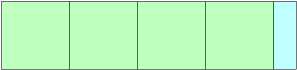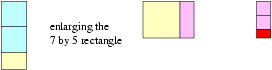# Euclid's Algorithm I

##### Age 16 to 18

Published 1999 Revised 2013

How can we solve equations like $13x+29y=42$ or $2x+4y=13$ with the solutions $x$ and $y$ being integers? Equations with integer solutions are called Diophantine equations after Diophantus who lived about 250 AD but the methods described here go back to Euclid (about 300 BC) and earlier. When people hear the name Euclid they think of geometry but the algorithm described here appeared as Proposition 2 in Euclid's Book 7 on Number Theory.

First we notice that $13x+29y=42$ has many solutions, for example $x=1$, $y=1$ and $x=30$, $y=-12$. Can you find others (it has infinitely many solutions)? We also notice that $2x+4y=13$ has no solutions because $2x+4y$ must be even and $13$ is odd. Can you find another equation that has no solutions?

If we can solve $3x+5y=1$ then we can also solve $3x+5y=456$. For example, $x=2$ and $y=-1$ is a solution of the first equation, so that $x=2\times 456$ and $y=-1\times 456$ is a solution of the second equation. The same argument works if we replace $456$ by any other number, so that we only have to consider equations with $1$ on the right hand side, for example $P x+Q y=1$. However if $P$ and $Q$ have a common factor $S$ then $P x+Q y$ must be a multiple of $S$ so we cannot have a solution of $P x+Q y=1$ unless $S=1$. This means that we should start by considering equations $P x+Q y=1$ where $P$ and $Q$ have no common factor.

Let us consider the example $83x+19y=1$. There is a standard method, called Euclid's Algorithm, for solving such equations. It involves taking the pair of numbers $P=83$ and $Q=19$ and replacing them successively by other pairs $(P_k,Q_k)$. We illustrate this by representing each pair of integers $(P_k,Q_k)$ by a rectangle with sides of length $P_k$ and $Q_k$.

Draw an $83$ by $19$ rectangle and mark off $4$ squares of side $19$, leaving a $19$ by $7$ rectangle.This diagram represents the fact that

$83=4\times 19+7$

In a few steps we shall split this rectangle into 'compartments' to illustrate the whole procedure for solving this equation. We repeat this process using the $19$ by $7$ rectangle to obtain two squares of side $7$, and a $7$ by $5$ rectangle. Next, the $7$ by $5$ rectangle splits into a square of side $5$, and a $5$ by $2$ rectangle.The $5$ by $2$ rectangle splits into two squares of side $2$, and a $2$ by $1$ rectangle. The $2$ by $1$ rectangle splits into two squares of side $1$ with nothing left over and the process finishes here as there is no residual rectangle. These diagrams illustrate the following equations:

\begin{eqnarray} 83 & = & 4\times 19 & + & 7\\ 19 & = & 2\times 7 & + & 5\\ 7 & = & 1\times 5 & + & 2\\ 5 & = & 2\times 2 & + & 1\\ 2 & = & 2\times 1 & + & 0 \end{eqnarray}

To find the solution we use the last non-zero remainder, namely $1=5-2$

and successively substitute the remainders from the other equations until we get back to the first one giving a combination of the two original values $P=83$ and $Q=19$. The method in this example has the following steps with the remainders given in square brackets.

\begin{eqnarray} 1 & = & -2\\ & = & -2(-)\\ & = & -2+3\\ & = & -2+3(19-2)\\ & = & (3\times 19)-8\\ & = & (3\times 19)-8(83-(4\times 19))\\ & = & (-8\times 83)+(35\times 19). \end{eqnarray}

Thus a solution of $83x+19y=1$ is $x=-8$ and $y=35$.

Can you find a solution of $83x+19y=7$?

Can you now find a solution of $827x+191y=2$? You should first solve the equation $827x+191y=1$ (using the computer if you wish).